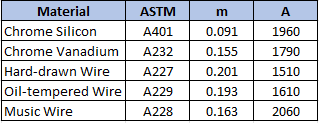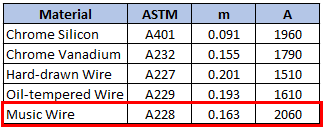## Tensile Strength for Springs

A music wire with a diameter of 7.25 mm will be used as a spring material in a piano. Calculate the minimum tensile strength of the wire.Hint
For spring materials, the minimum tensile strength for common spring steels can be determined from:
$$S_{ut}=\frac{A}{d^{m}}$$$where $$S_{ut}$$ is the tensile strength in MPa, and $$d$$ is the wire diameter in millimeters. Hint 2 $$A$$ and $$m$$ can be found in the below table:For spring materials, the minimum tensile strength for common spring steels can be determined from: $$S_{ut}=\frac{A}{d^{m}}$$$
where $$S_{ut}$$ is the tensile strength in MPa, $$d$$ is the wire diameter in millimeters, and $$A$$ and $$m$$ can be found in the below table:Since the problem is asking for the minimum tensile strength of a music wire:
$$S_{ut}=\frac{2060}{(7.25mm)^{0.163}}=\frac{2060}{1.381}=1492\: MPa$$\$
1492 MPa